# I.E. Irodov Solutions on Motion of Charged Particles in Electric and Magnetic Fields

Motion of Charged Particles in Electric and Magnetic Fields is an important chapter in Physics that mainly deals with electromagnetic induction. Solving I.E. Irodov book problems is one of the best ways to prepare for the JEE Main exam to score high. The problems asked here are mainly based on current in a circuit, voltage, movement of charge and so on.

The numerical are mainly based on the popular movement of charge aimed at determining the flow of current in the electrical circuit. This movement also aims at explaining the variation of electrical and magnetic fields. I.E. Irodov solutions help the students to analyse which law can be applied to solve the problems. The problems given here focus on the variation of magnetic field, how it is applied and its changes with the respective materials. Access I.E. Irodov book Chapter 3.7 problems here.

### I.E. Irodov Solutions on Polarization of Light

1. At the moment t = 0, an electron leaves one plate of a parallel-plate capacitor with a negligible velocity. An accelerating voltage, varying as V = at, where a = 100 V/s, is applied between the plates. The separation between the plates is l = 5.0 cm. What is the velocity of the electron at the moment it reaches the opposite plate?

Solution:

1. Let the electron leave the negative plate of the capacitor at time t = 0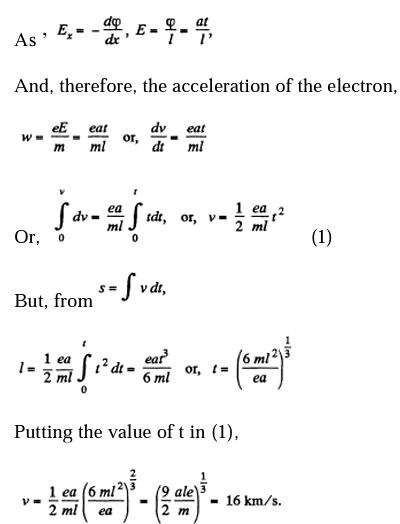2. A proton accelerated by a potential difference V gets into the uniform electric field of a parallel-plate capacitor whose plates extend over a length of 1 in the motion direction. The field strength varies with time as E = at, where a is a constant. Assuming the proton to be non-relativistic, find the angle between the motion directions of the proton before and after its flight through the capacitor; the proton gets in the field at the moment t = 0. The edge effects are to be neglected.

Solution:

1. The electric field inside the capacitor varies with time as E = at.

Hence, electric force on the proton, F = eat

And subsequently, acceleration of the proton,

\omega =\frac{eat}{m}

Now, if t is the time elapsed during the motion of the proton between the plates, t=\frac{l}{v_{\mathbb{I}}} as no acceleration is effective in this direction.

{v_{\mathbb{I}}} is velocity along the length of the plate.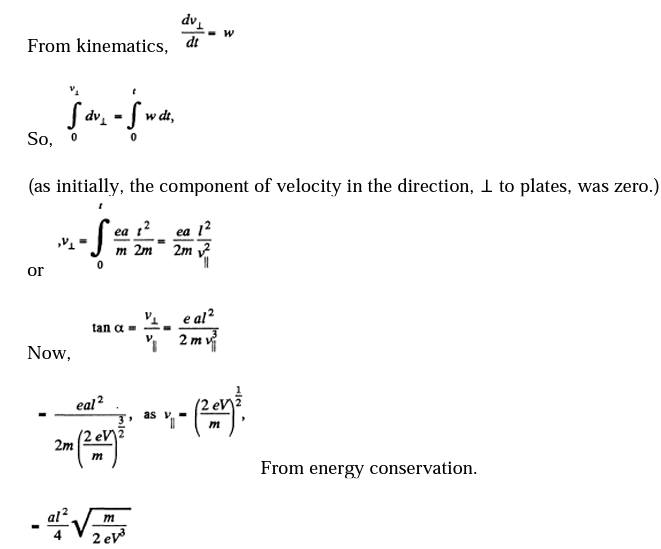3. A particle with a specific charge qlm moves rectilinearly due to an electric field E = E0 — ax, where a is a positive constant, x is the distance from the point where the particle was initially at rest. Find:

(a) the distance covered by the particle till the moment it came to a standstill;

(b) the acceleration of the particle at that moment.

Solution:

1. The equation of motion is,

\frac{dv}{dt}=v\frac{dv}{dx}=\frac{q}{m}(E_{o}-ax)

On integrating we get

\frac{1}{2}v^{2}-\frac{q}{m}(E_{0}x-\frac{1}{2}ax^{2})= constant

But initially v = 0 when x = 0, so “constant” = 0

Thus,

v^{2}=\frac{2q}{m}(E_{0}x-\frac{1}{2}ax^{2})

Thus. v = 0, again for

x=x_{m}=\frac{2E_{0}}{a}

Thus corresponding acceleration is,

\left ( \frac{dv}{dt} \right )_{x_{m}}=\frac{q}{m}(E_{0}-2E_{0})=-\frac{qE_{o}}{m}

4. An electron starts moving in a uniform electric field of strength E = 10 kV/cm. How soon after the start will the kinetic energy of the electron become equal to its rest energy?

Solution:

1. From the law of relativistic conservation of energy

\frac{m_{0}C^{2}}{\sqrt{1-\left ( \frac{v^{2}}{c^{2}} \right )}}-eEx=m_{0}C^{2}

As the electron is at rest (v = 0 for x = 0) initially.

Thus clearly T = eEx.

On the other hand,

\sqrt{1-\left ( \frac{v^{2}}{c^{2}} \right )}=\frac{m_{0}C^{2}}{m_{0}C^{2}+ eEx}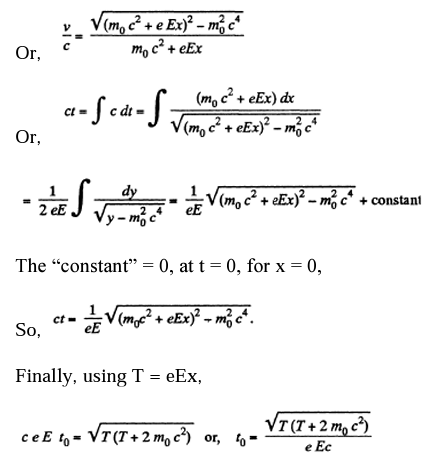5. Determine the acceleration of a relativistic electron moving along a uniform electric field of strength E at the moment when its kinetic energy becomes equal to T.

Solution:

1. As before, T = Ex

Now in linear motion,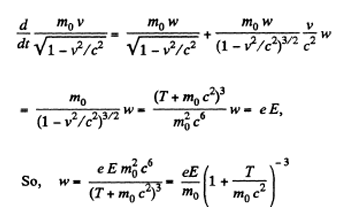6. At the moment t = 0 a relativistic proton flies with a velocity v, into the region where there is a uniform transverse electric field of strength E, with v0 ⊥ E. Find the time dependence of

(a) the angle θ between the proton's velocity vector v and the initial direction of its motion

(b) the projection vx of the vector v on the initial direction of motion

Solution:

1. The equations are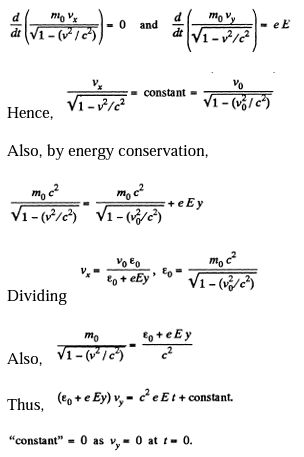Thus,

(ceET)^{2}=(eyE)^{2}+2\varepsilon _{o}eEy+\varepsilon _{0}^{2}-\varepsilon _{0}^{2}

(ceET)^{2}=\sqrt{(\varepsilon _{o}+eEy)^{2}-\varepsilon _{0}^{2}}

\varepsilon _{o}+eEy=\sqrt{\varepsilon _{o}^{2}+C^{2}e^{2}E^{2}t^{2}}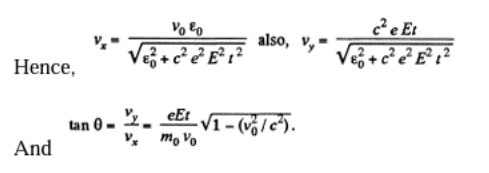7. A proton accelerated by a potential difference V = 500kV flies through a uniform transverse magnetic field with induction B = 0.51T. The field occupies a region of space d =10 cm in thickness (Figure). Find the angle α through which the proton deviates from the initial direction of its motion.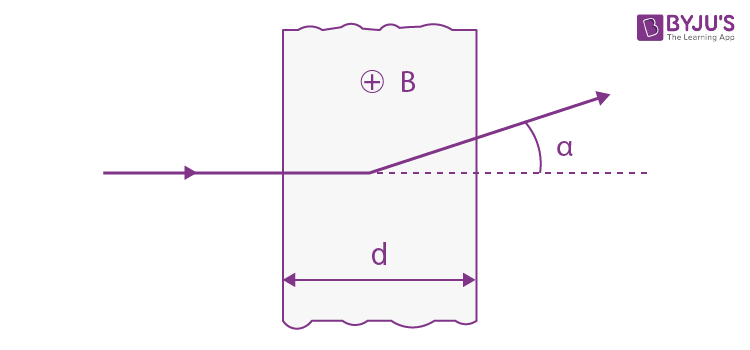Solution:

1. From the figure

R=\frac{mv}{qb}

where v is the velocity of the particle, when it enters into the field. From initial condition of the problem,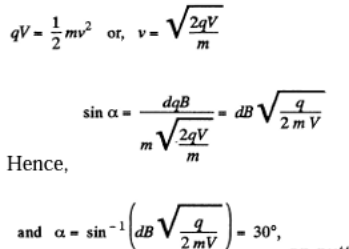(on substituting values, we get above result)

8. A charged particle moves along a circle of radius r = 100 mm in a uniform magnetic field with induction B = 10.0 mT. Find its velocity and period of revolution if that particle is

(a) a non-relativistic proton;

(b) a relativistic electron

Solution:

1. (a)For motion along a circle, the magnetic force acted on the particle, will provide the centripetal force, necessary for its circular motion.

I.e \frac{mv^{2}}{r}=evB\Rightarrow v=\frac{eBR}{m}

And the period of revolution

T=\frac{2\pi }{\omega}=\frac{2\pi R }{v}=\frac{2\pi m}{eB}

(b) Generally \frac{d\vec{p}}{dt}=\vec{F}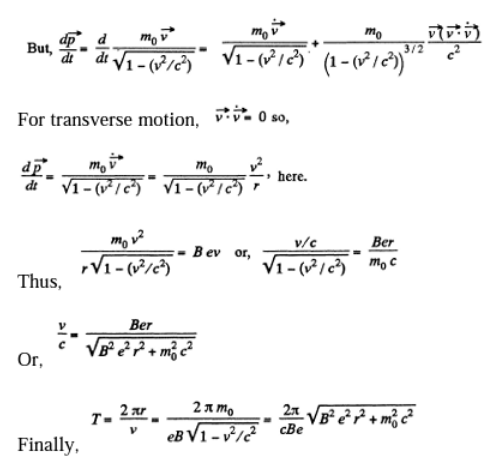9. A relativistic particle with charge q and rest mass m0 moves along a circle of radius r in a uniform magnetic field of induction B. Find:

(a) the modulus of the particle's momentum vector;

(b) the kinetic energy of the particle;

(c) the acceleration of the particle.

Solution:

1. (a) As before, p = B qr

(b)T=\sqrt{C^{2}p^{2}+m_{o}C^{4}}=\sqrt{C^{2}B^{2}q^{2}r^{2}+m_{0}^{2}C^{4}}

(c)\omega =\frac{v^{2}}{r}=\frac{c^{2}}{r(1+(\frac{m_{0}C}{Bqr})^{2}}

Using the result for v from the previous problem.

10. Up to what values of kinetic energy does the period of revolution of an electron and a proton in a uniform magnetic field exceed that at non-relativistic velocities by η = 1.0 % ?

Solution:

1.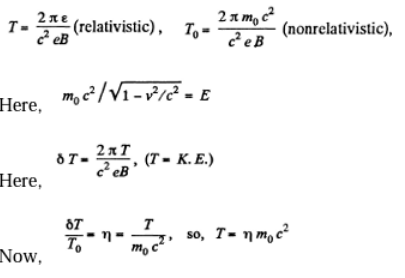11. An electron accelerated by a potential difference V = 1.0 kV moves in a uniform magnetic field at an angle α = 30° to the vector B whose modulus is B = 29 mT. Find the pitch of the helical trajectory of the electron.

Solution:

1. T=ev=½ mv2

The given potential difference is not large enough to cause significant deviations from the nonrelativistic formula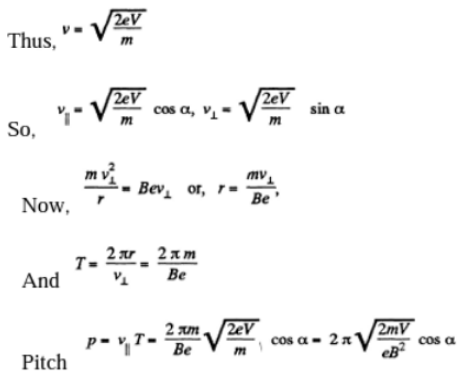12. A slightly divergent beam of non-relativistic charged particles accelerated by a potential difference V propagates from a point A along the axis of a straight solenoid. The beam is brought into focus at a distance l from the point A at two successive values of magnetic induction B1 and B2. Find the specific charge q/m of the particles.

Solution:

1. The charged particles will traverse a helical trajectory and will be focussed on the axis after traversing a number of turms. Thus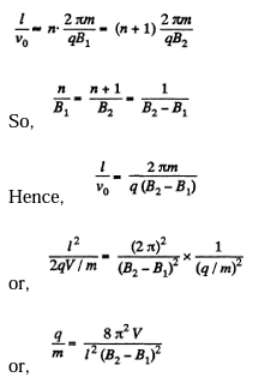13. A non-relativistic electron originates at a point A lying on the axis of a straight solenoid and moves with velocity v at an angle α to the axis. The magnetic induction of the field is equal to B. Find the distance r from the axis to the point on the screen into which the electron strikes. The screen is oriented at right angles to the axis and is located at a distance l from point A.

Solution:

1. Let us take point A as the origin O and the axis of the solenoid as the z-axis. At an arbitrary moment of time let us resolve the velocity of electron into its two rectangular components, \vec{V_{\mathbb{I}}} along the axis\vec{V_{1}} to the axis of the solenoid. We know the magnetic force does no work, so the kinetic energy as well as the speed of the electron \left | \vec{V_{1}}\right | will remain constant in the x-y plane. Thus \vec{V_{\mathbb{I}}} will remain constant to \vec{B}

Thus at t=t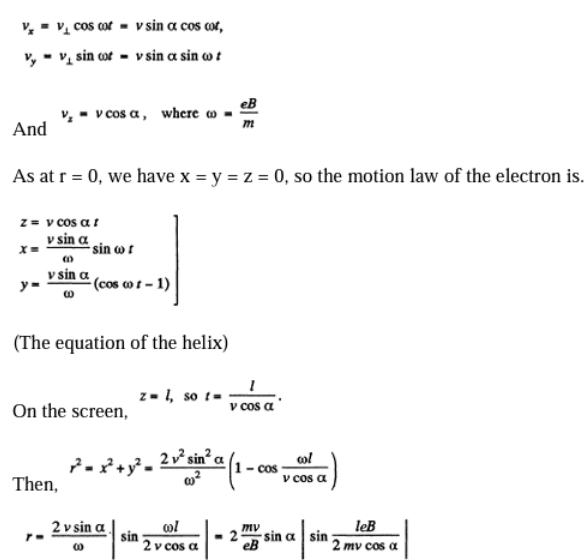14. From the surface of a round wire of radius a carrying a direct current I an electron escapes with a velocity vo perpendicular to the surface. Find what will be the maximum distance of the electron from the axis of the wire before it turns back due to the action of the magnetic field generated by the current.

Solution:

1. Choose the wire along the z-axis, and the initial direction of the electron, along the x-axis. Then the magnetic field in the x - z plane is along the y - axis and outside the wire it is,

B=B^{y}=\frac{\mu _{0}I}{2\pi x}

(Bx=Bz=0 if y=0)

The motion must be confined to the x - z plane. Then the equations of motion are,

\frac{d}{dt}mv_{z}=-ev_{z}B_{y}

\frac{d}{dt}mv_{z}=+ev_{x}B_{y}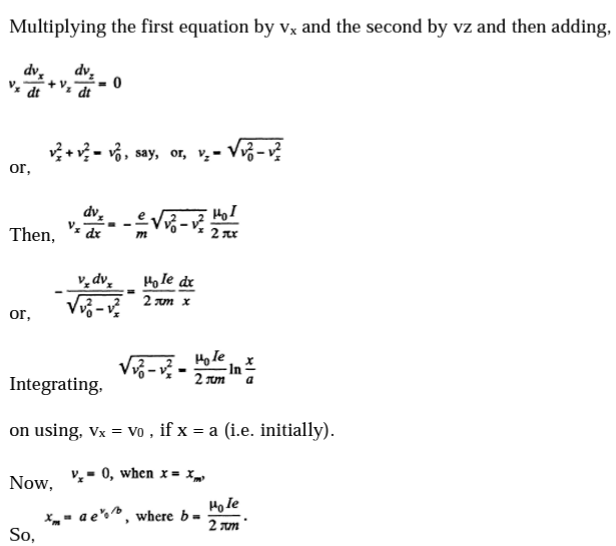15. A non-relativistic charged particle flies through the electric field of a cylindrical capacitor and gets into a uniform transverse magnetic field with induction B (Figure). In the capacitor, the particle moves along the arc of a circle, in the magnetic field, along a semi-circle of radius r. The potential difference applied to the capacitor is equal to V, the radii of the electrodes are equal to a and b, with a < b. Find the velocity of the particle and its specific charge q/m.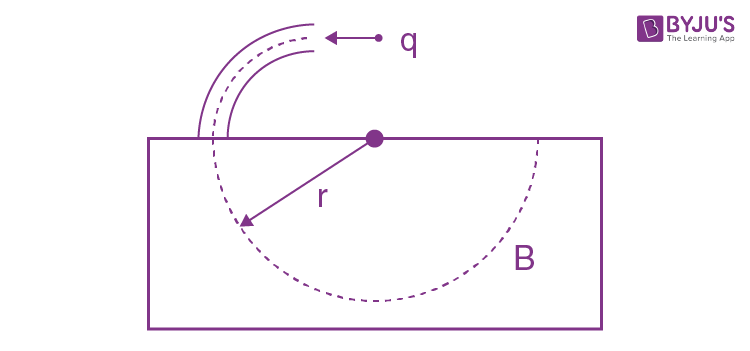Solution:

1. Inside the capacitor, the electric field follows a\frac{1}{r} law and so the potential can be written as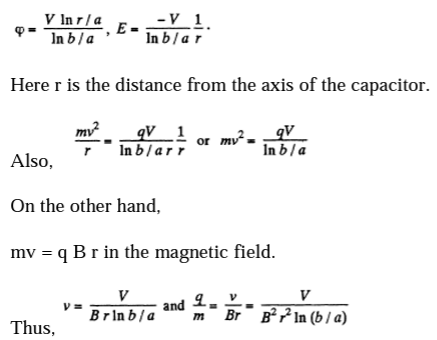16. Uniform electric and magnetic fields with strength E and induction B respectively are directed along the y axis (Fig. 3.101). A particle with a specific charge q/m leaves the origin O in the direction of the x-axis with an initial non-relativistic velocity v 0. Find:

(a)The coordinate ynof the particle when it crosses the y axis for the nth time;

(b) the angle α between the particle's velocity vector and the y axis at that moment

Solution:

1.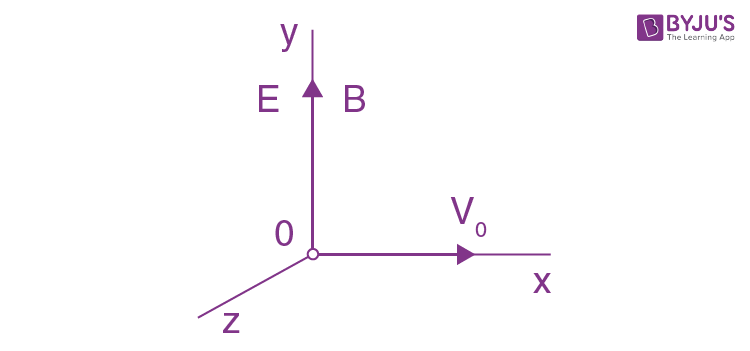The equations of motion are,

m\frac{dv_{x}}{dt}=-qvB_{z}

m\frac{dv_{y}}{dt}=-qE

m\frac{dv_{z}}{dt}=-qv_{x}B

These equations can be solved easily,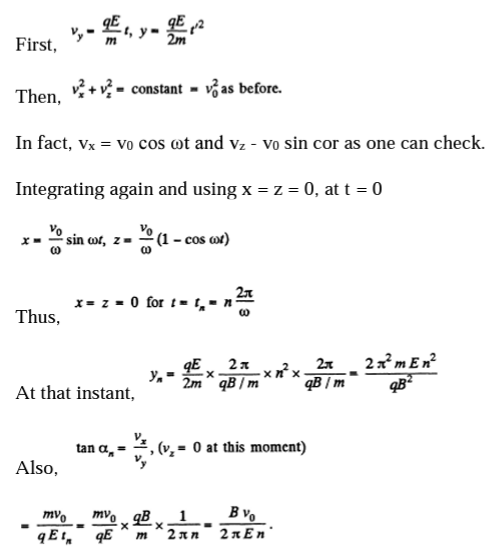17. A narrow beam of identical ions with specific charge q/m, possessing different velocities, enters the region of space, where there are uniform parallel electric and magnetic fields with strength E and induction B, at the point O (figure). The beam direction coincides with the x-axis at the point O. A plane screen-oriented at right angles to the x- axis is located at a distance l from the point O. Find the equation of the trace that the ions leave on the screen. Demonstrate z<<1 it is the equation of a parabola.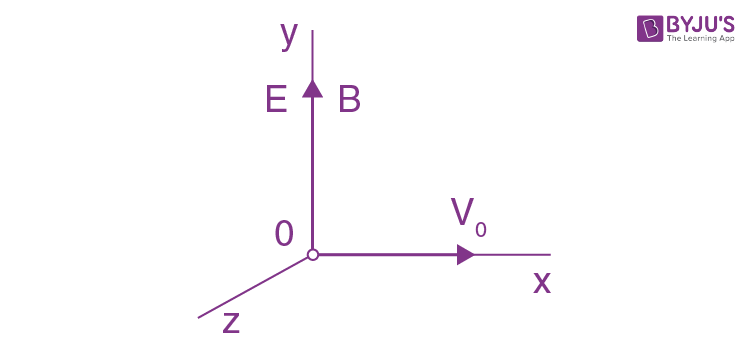Solution:

1. The equation of the trajectory is

x=\frac{v_{o}}{w}\sin \omega t, z=\frac{v_{o}}{w}(1-\cos \omega t), y=\frac{qe}{2m}t^{2}

Now on the screen x = l, so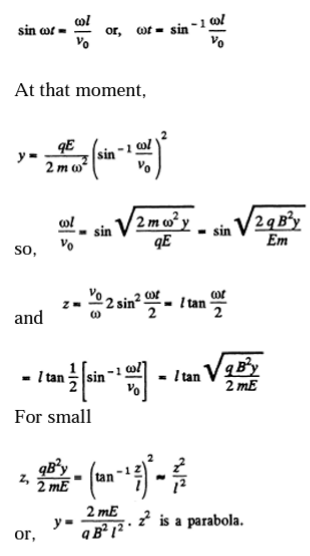18. A non-relativistic proton beam passes without deviation through the region of space where there are uniform transverse mutually perpendicular electric and magnetic fields with E = 120 kV/m and B = 50 mT. Then the beam strikes a grounded target. Find the force with which the beam acts on the target if the beam current is equal to I = 0.80 mA.

Solution:

1. In crossed field,

eE=evB\Rightarrow v=\frac{E}{B}

Then, F = force exerted on the plate

\frac{I}{e}\times m\frac{E}{B}=\frac{mIE}{eB}

19. Non-relativistic protons move rectilinearly in the region of space where there are uniform mutually perpendicular electric and magnetic fields with E = 4.0 kV/m and B = 50 mT. The trajectory of the protons lies in the plane xz (Figure) and forms an angle φ = 30° with the x-axis. Find the pitch of the helical trajectory along which the protons will move after the electric field is switched off.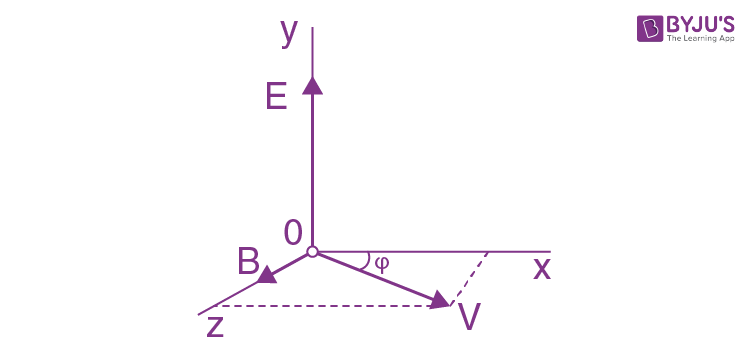Solution:

1. When the electric field is switched off, the path followed by the particle will be helical and pitch

\Delta l=v_{\mathbb{I}}T where \v_{\mathbb{I}} is the velocity of the particle, parallel to \vec{B} and T the time period of solution.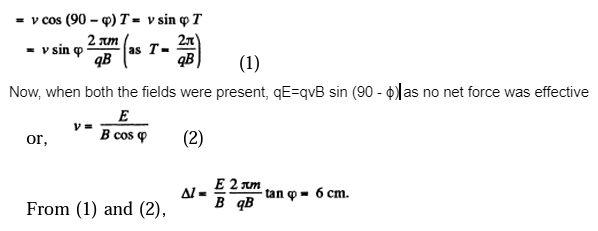20. A beam of non-relativistic charged particles moves without deviation through the region of space A (Figure) where there are transverse mutually perpendicular electric and magnetic fields with strength E and induction B. When the magnetic field is switched off, the trace of the beam on the screen S shifts by Δx. Knowing the distances a and b, find the specific charge q/m of the particles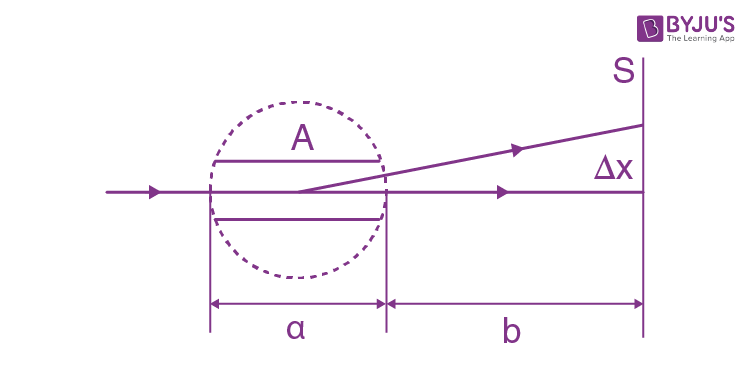Solution:

1. When there is no deviation

-q\vec{E}=q(\vec{v}\times \vec{B})

or, in scalar from,

E=vB \left ( \vec{V}\perp \vec{B}\right ) \Rightarrow v=\frac{E}{B} (1)

Now, when the magnetic field is switched on, let the deviation in the field be x. Then

x=\frac{l}{2}(\frac{qvB}{m})t^{2}

where t is the time required to pass through this region,

t=a/v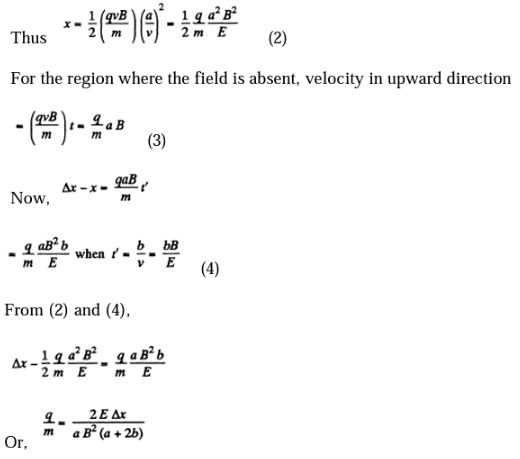21. A particle with specific charge qim moves in the region of space where there are uniform mutually perpendicular electric and magnetic fields with strength E and induction B, (Figure). At the moment t = 0 the particle was located at the point O and had zero velocity. For the nonrelativistic case find:

(a) the law of motion x (t) and y (t) of the particle; the shape of the trajectory;

(b) the length of the segment of the trajectory between two nearest points at which the velocity of the particle turns into zero;

(c) the mean value of the particles, velocity vector projection on the x-axis(the drift velocity).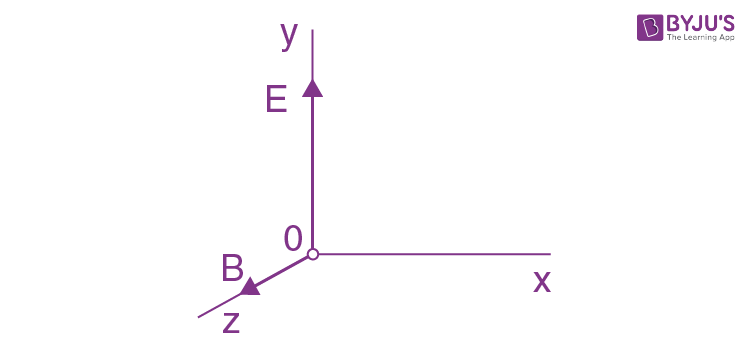Solution:

1. (a)The equation of motion is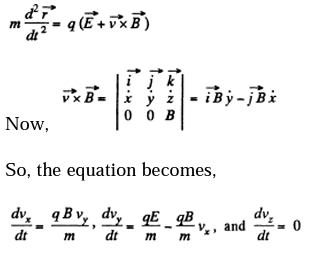Here v_{x}=\dot{x},v_{y}=\dot{y},v_{z}=\dot{z}

The last equation is easy to integrate;

vz = constant = 0,

since vz is zero initially.

Thus integrating again, z = constant = 0, and motion is confined to the x - y plane.

We now multiply the second equation by i and add it to the first equation.

\varepsilon =v_{x}-iv_{y}

We get the equation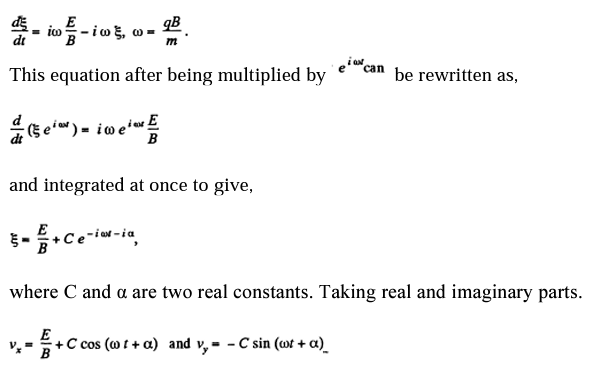Since Vy=0 when t=0 we can take α=0 then Vx=0 at t=o gives C=-E/B and we get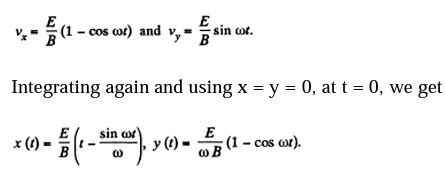This is the equation of a cycloid

(b) The velocity is zero when \omega t-2n\pi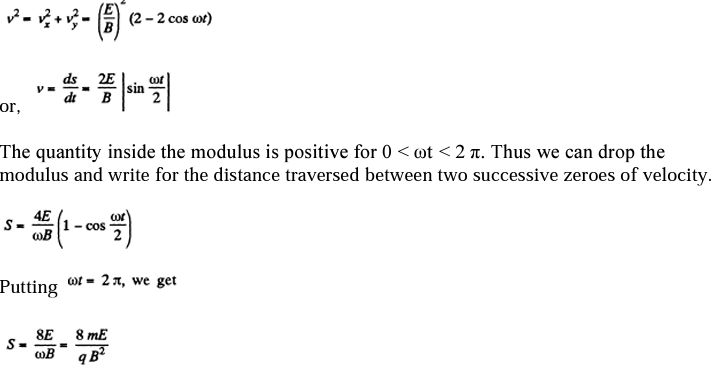(c) The drift velocity is in the x-direction and has the magnitude

<V_{x}>=<\frac{E}{B}1-\cos \omega t>=\frac{E}{B}